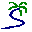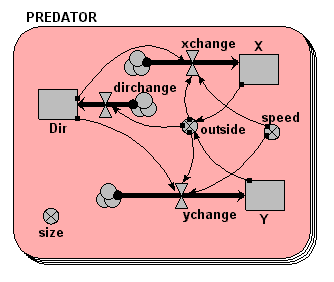# Model entry

Simple model of animal movement - Model catalogue - Simulistics.com

 Search Simulistics Model catalogue Listed by keyword Listed by ID Listed by title Listed by date added

# Simple model of animal movement

Model : hunter1
Simile version : 3.1+
Keywords : Movement ; Individual-based ; Spatial modelling ; Fixed-membership submodel ; System Dynamics ;

## Description

This models the partly-random movement of an animal on a plane surface.

We use three state variables: one for the animal�s x-coordinate, one for its y coordinate, and one for the direction it is currently facing.

Each time step, the animal changes its direction slightly to the left or right (by selecting a change-of-direction value randomly from a range of angles), then moves ahead that amount. We need a bit of trigonometry to work out how much the x and y values change knowing the new direction and the distance it travels each time step (i.e. its speed).

The model includes an edge detector, to stop the animal wandering off the screen.

## Files

### Model file

Click on the icon to download the model file. (You will need Simile to examine and run the model. A free evaluation version is available from the products page.)

Some browsers may attempt to display the model file, rather than open it in Simile; in this case, use the browser back button to return to this page, and use the context menu (invoked by right-clicking on the link) to save the target file to disk.hunter1.sml

## Diagram## Equations

```Equations in PREDATOR
Compartments:
X:   initial value=rand(30,70)
Dir: initial value=rand(0,6.28)
Y:   initial value==rand(30,70)

Flows:
xchange   = (if outside==1 then speed*cos(dir+3.14)else speed*cos(dir))
dirchange = (if outside==1 then 3.14/dt(1)else rand(-0.4,0.4)/dt(1))
ychange   = (if outside==1 then speed*sin(dir+3.14)else speed*sin(dir))

Variables:
speed     = 5
size      = 3
outside   = (if (x<0;x>100;y<0;y>100)then 1 else 0)
```